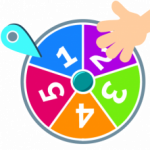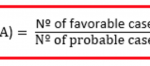Smartick is a fun way to learn math!# I want to learn about: Statistics & Probability

Learn statistics and probability with Smartick. Exercises, tutorials, problems, and teaching resources about statistics and probability for elementary aged children. Here you will find posts about statistics and probability to make learning mathematics easier and more fun.

Apr09

## Probability: What It Is and How to Calculate It with ExamplesIn this post, we are going to learn quite a few things about probability: what it is, what it’s used for, and how it is calculated, as well as some examples that we could find in our daily life. What is Probability? One of the most special characteristics humans possess that sets us apart from […]

Sep22

## Learn Probability Problems and ExamplesIn today’s post, we are going to solve a probability problem that appeared in Madrid’s 2008 Standardized Elementary Testing Exam (CDI): Let’s start by reviewing some probability concepts. Sample space: The sample space refers to all of the possible outcomes of a random experiment and it’s often represented by E (or as omega, Ω, from […]